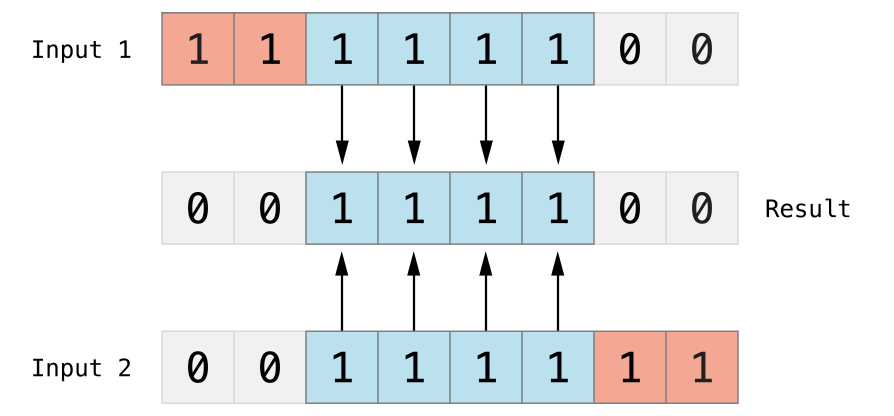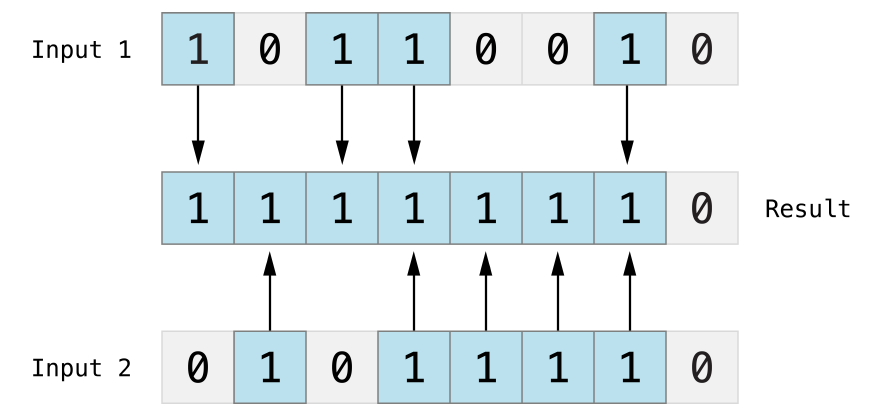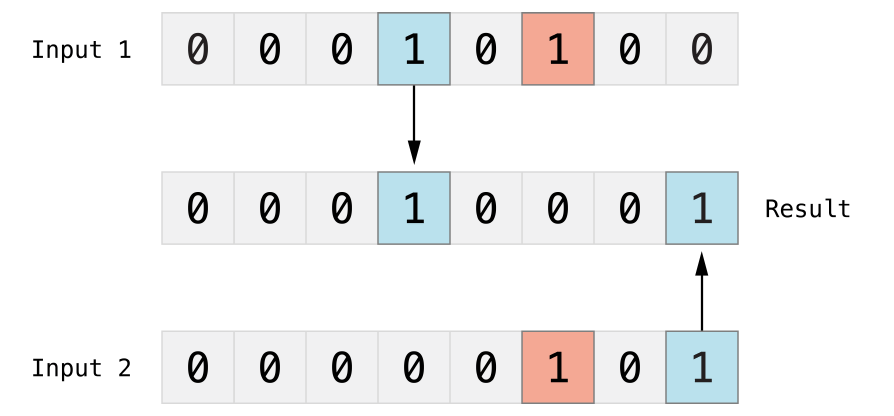Swift 运算符

Swift 提供了以下几种运算符：

• 算术运算符
• 比较运算符
• 逻辑运算符
• 位运算符
• 赋值运算符
• 区间运算符
• 其他运算符

算术运算符

+ 加号 A + B 结果为 30

* 乘号 A * B 结果为 200
/ 除号 B / A 结果为 2
% 求余 B % A 结果为 0

实例

```import Cocoa

var A = 10
var B = 20

print("A + B 结果为：\(A + B)")
print("A - B 结果为：\(A - B)")
print("A * B 结果为：\(A * B)")
print("B / A 结果为：\(B / A)")
A += 1   // 类似 A++
print("A += 1 后 A 的值为 \(A)")
B -= 1   // 类似 B--
print("B -= 1 后 B 的值为 \(B)")
```

```A + B 结果为：30
A - B 结果为：-10
A * B 结果为：200
B / A 结果为：2
A += 1 后 A 的值为 11
B -= 1 后 B 的值为 19
```

比较运算符

== 等于 (A == B) 为 false。
!= 不等于 (A != B) 为 true。
> 大于 (A > B) 为 false。
< 小于 (A < B) 为 true。
>= 大于等于 (A >= B) 为 false。
<= 小于等于 (A <= B) 为 true。

实例

```import Cocoa

var A = 10
var B = 20

print("A == B 结果为：\(A == B)")
print("A != B 结果为：\(A != B)")
print("A > B 结果为：\(A > B)")
print("A < B 结果为：\(A < B)")
print("A >= B 结果为：\(A >= B)")
print("A <= B 结果为：\(A <= B)")
```

```A == B 结果为：false
A != B 结果为：true
A > B 结果为：false
A < B 结果为：true
A >= B 结果为：false
A <= B 结果为：true
```

逻辑运算符

&& 逻辑与。如果运算符两侧都为 TRUE 则为 TRUE。 (A && B) 为 false。
|| 逻辑或。 如果运算符两侧至少有一个为 TRUE 则为 TRUE。 (A || B) 为 true。
! 逻辑非。布尔值取反，使得true变false，false变true。 !(A && B) 为 true。

```import Cocoa

var A = true
var B = false

print("A && B 结果为：\(A && B)")
print("A || B 结果为：\(A || B)")
print("!A 结果为：\(!A)")
print("!B 结果为：\(!B)")
```

```A && B 结果为：false
A || B 结果为：true
!A 结果为：false
!B 结果为：true
```

位运算符

p q p & q p | q p ^ q
0 0 0 0 0
0 1 0 1 1
1 1 1 1 0
1 0 0 1 1

```A = 0011 1100

B = 0000 1101
```

& 按位与。按位与运算符对两个数进行操作，然后返回一个新的数，这个数的每个位都需要两个输入数的同一位都为1时才为1。(A & B) 结果为 12, 二进制为 0000 1100
| 按位或。按位或运算符|比较两个数，然后返回一个新的数，这个数的每一位设置1的条件是两个输入数的同一位都不为0(即任意一个为1，或都为1)。(A | B) 结果为 61, 二进制为 0011 1101
^ 按位异或. 按位异或运算符^比较两个数，然后返回一个数，这个数的每个位设为1的条件是两个输入数的同一位不同，如果相同就设为0。(A ^ B) 结果为 49, 二进制为 0011 0001
~ 按位取反运算符~对一个操作数的每一位都取反。(~A ) 结果为 -61, 二进制为 1100 0011 in 2's complement form.
<< 按位左移。左移操作符（

A << 2 结果为 240, 二进制为 1111 0000
>> 按位右移。右移操作符（A >> 2 结果为 15, 二进制为 0000 1111

```import Cocoa

var A = 60  // 二进制为 0011 1100
var B = 13 // 二进制为 0000 1101

print("A&B 结果为：\(A&B)")
print("A|B 结果为：\(A|B)")
print("A^B 结果为：\(A^B)")
print("~A 结果为：\(~A)")
```

```A&B 结果为：12
A|B 结果为：61
A^B 结果为：49
~A 结果为：-61
```

赋值运算

= 简单的赋值运算，指定右边操作数赋值给左边的操作数。 C = A + B 将 A + B 的运算结果赋值给 C
+= 相加后再赋值，将左右两边的操作数相加后再赋值给左边的操作数。 C += A 相当于 C = C + A
-= 相减后再赋值，将左右两边的操作数相减后再赋值给左边的操作数。 C -= A 相当于 C = C - A
*= 相乘后再赋值，将左右两边的操作数相乘后再赋值给左边的操作数。 C *= A 相当于 C = C * A
/= 相除后再赋值，将左右两边的操作数相除后再赋值给左边的操作数。 C /= A 相当于 C = C / A
%= 求余后再赋值，将左右两边的操作数求余后再赋值给左边的操作数。 C %= A is equivalent to C = C % A
<<= 按位左移后再赋值 C <<= 2 相当于 C = C << 2
>>= 按位右移后再赋值 C >>= 2 相当于 C = C >> 2
&= 按位与运算后赋值 C &= 2 相当于 C = C & 2
^= 按位异或运算符后再赋值 C ^= 2 相当于 C = C ^ 2
|= 按位或运算后再赋值 C |= 2 相当于 C = C | 2

```import Cocoa

var A = 10
var B = 20
var C = 100

C = A + B
print("C 结果为：\(C)")

C += A
print("C 结果为：\(C)")

C -= A
print("C 结果为：\(C)")

C *= A
print("C 结果为：\(C)")

C /= A
print("C 结果为：\(C)")

//以下测试已注释，可去掉注释测试每个实例
/*
C %= A
print("C 结果为：\(C)")

C <<= A
print("C 结果为：\(C)")

C >>= A
print("C 结果为：\(C)")

C &= A
print("C 结果为：\(C)")

C ^= A
print("C 结果为：\(C)")

C |= A
print("C 结果为：\(C)")
*/
```

```C 结果为：30
C 结果为：40
C 结果为：30
C 结果为：300
C 结果为：30
```

区间运算符

Swift 提供了两个区间的运算符。

```import Cocoa

print("闭区间运算符:")
for index in 1...5 {
print("\(index) * 5 = \(index * 5)")
}

print("半开区间运算符:")
for index in 1..<5 {
print("\(index) * 5 = \(index * 5)")
}
```

```闭区间运算符:
1 * 5 = 5
2 * 5 = 10
3 * 5 = 15
4 * 5 = 20
5 * 5 = 25

1 * 5 = 5
2 * 5 = 10
3 * 5 = 15
4 * 5 = 20
```

其他运算符

Swift 提供了其他类型的的运算符，如一元、二元和三元运算符。

• 一元运算符对单一操作对象操作（如`-a`）。一元运算符分前置运算符和后置运算符，前置运算符需紧跟在操作对象之前（如`!b`），后置运算符需紧跟在操作对象之后（例如c!）。备注：在Java／C没有类似c！的语法， 在Swift中用在Optional类型取值。
• 二元运算符操作两个操作对象（如`2 + 3`），是中置的，因为它们出现在两个操作对象之间。
• 三元运算符操作三个操作对象，和 C 语言一样，Swift 只有一个三元运算符，就是三目运算符（`a ? b : c`）。

```import Cocoa

var A = 1
var B = 2
var C = true
var D = false
print("-A 的值为：\(-A)")
print("A + B 的值为：\(A + B)")
print("三元运算：\(C ? A : B )")
print("三元运算：\(D ? A : B )")
```

```-A 的值为：-1
A + B 的值为：3

```

运算符优先级

• 指针最优，单目运算优于双目运算。如正负号。
• 先乘除（模），后加减。
• 先算术运算，后移位运算，最后位运算。请特别注意：1
• 逻辑运算最后计算

* & + - ! ~

* / %

+ -

>> <<

< > <= >=

== !=

&

^

|

&&

||

```import Cocoa

var A = 0

A = 2 + 3 * 4 % 5
print("A 的值为：\(A)")
```

```A 的值为：4
```

```2 + ((3 * 4) % 5)
```

`2 + (12 % 5)`

`2 + 2`